# Adding Straight Lines to a Plot in R Programming – abline() Function

`abline()` function in R Language is used to add one or more straight lines to a graph. The `abline()` function can be used to add vertical, horizontal or regression lines to plot.

Syntax:
abline(a=NULL, b=NULL, h=NULL, v=NULL, …)

Parameters:
a, b: It specifies the intercept and the slope of the line
h: specifies y-value for horizontal line(s)
v: specifies x-value(s) for vertical line(s)

Returns: a straight line in the plot

Example 1: To add a vertical line to the plot

 `# add line to square plot  ` `# first example : Add one line ` `plot``(cars) ` `abline``(v = 16, col = ``"darkgreen"``) ` ` `  `# second example : add 2 lines  ` `# addline to square plot  ` `# change line colors, sizes and types ` `plot``(cars) ` `abline``(v = ``c``(16, 22), col = ``c``(``"darkgreen"``, ``"blue"``), ` `                      ``lty = ``c``(1, 2), lwd = ``c``(1, 3)) ` ` `  `# third example ` `set.seed``(1200); mydata<-``rnorm``(180) ` `hist``(mydata, col=``"darkgreen"``) ` ` `  `# lwd=line width, lty =linetype  ` `abline``(v = ``mean``(mydata), col = ``"blue"``, lwd = 4, lty = 4)  `

Output: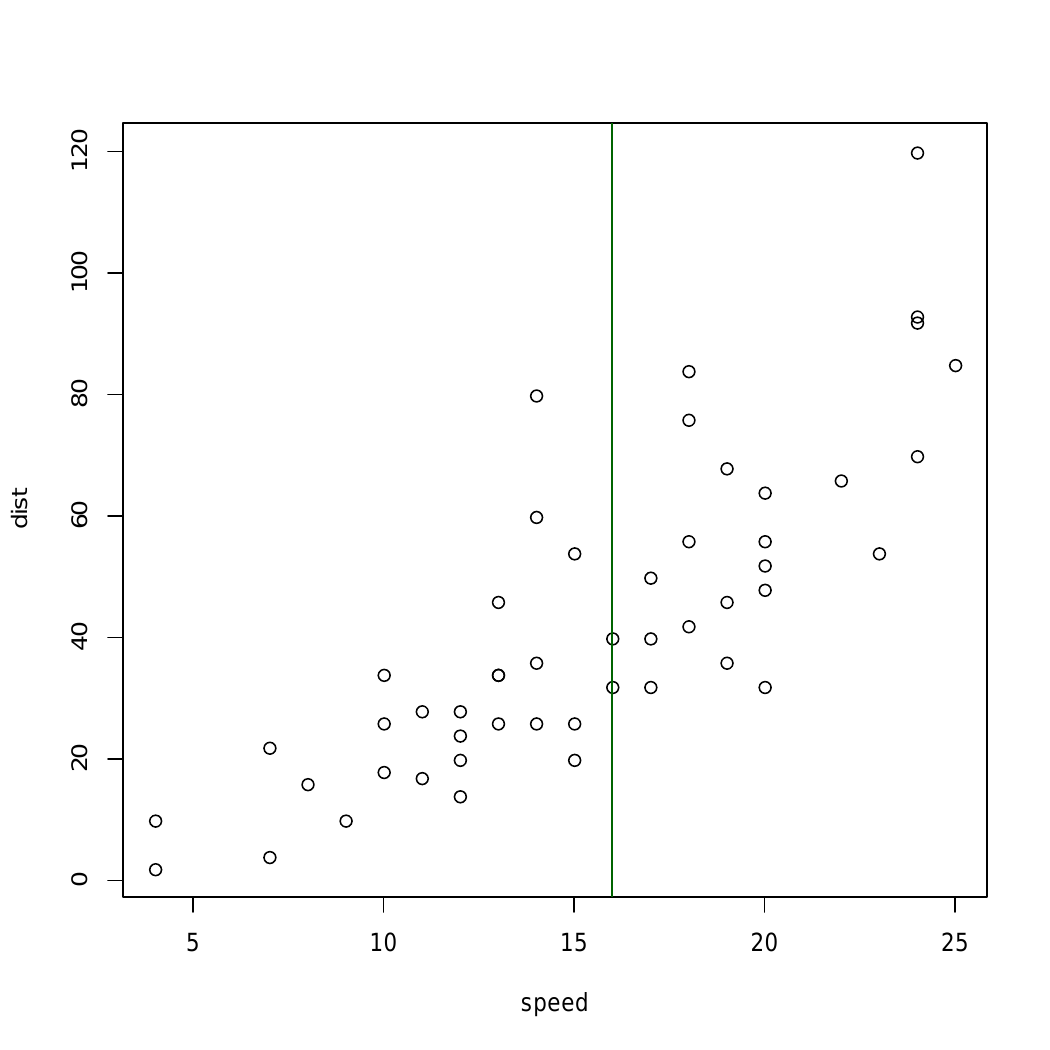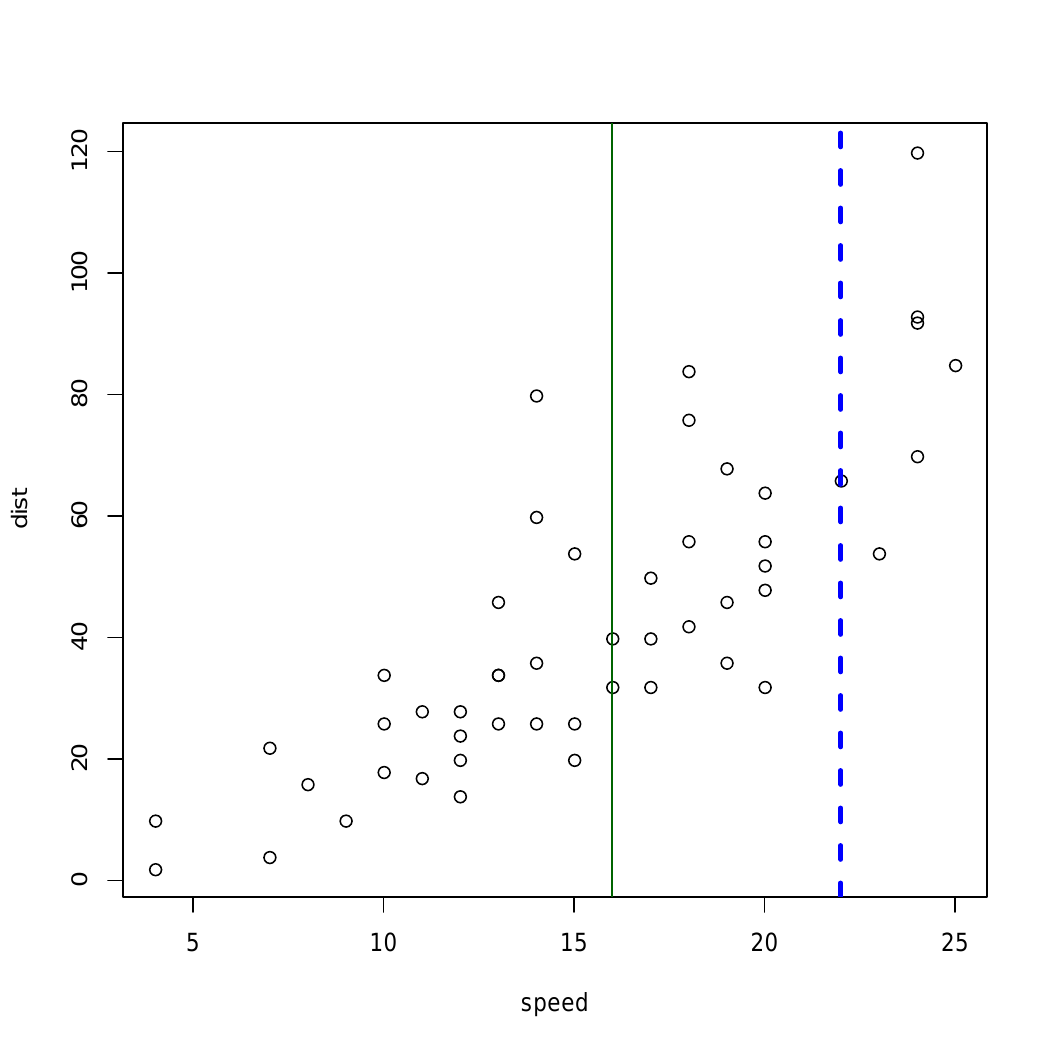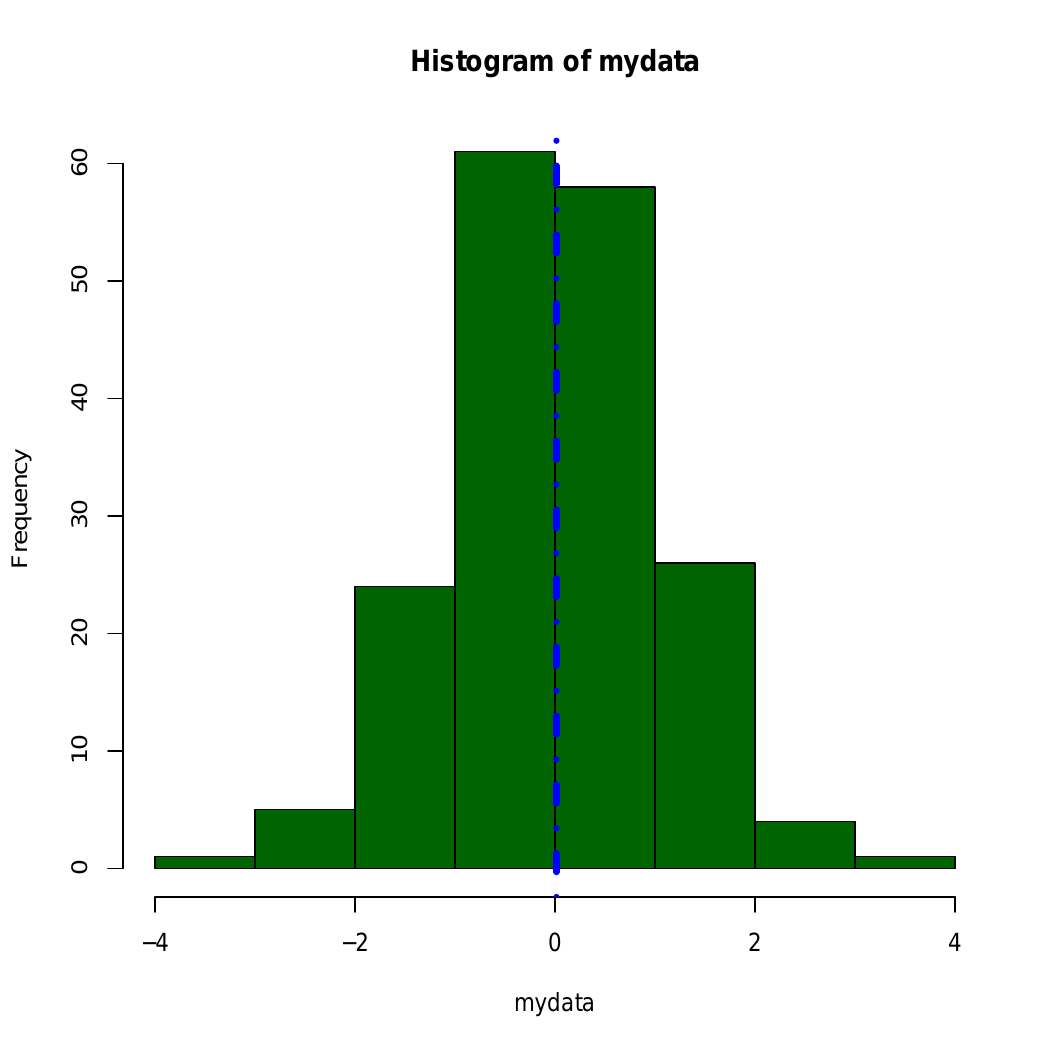Here, in above example straight line is added using abline() to different graphical plots

Example 2: To add a horizontal line

 `# R program to add a horizontal line ` `# to a plot ` ` `  `# Creating a plot ` `plot``(cars) ` ` `  `# Calling abline() function ` `abline``(h = 60, col = ``"darkgreen"``) `

Output: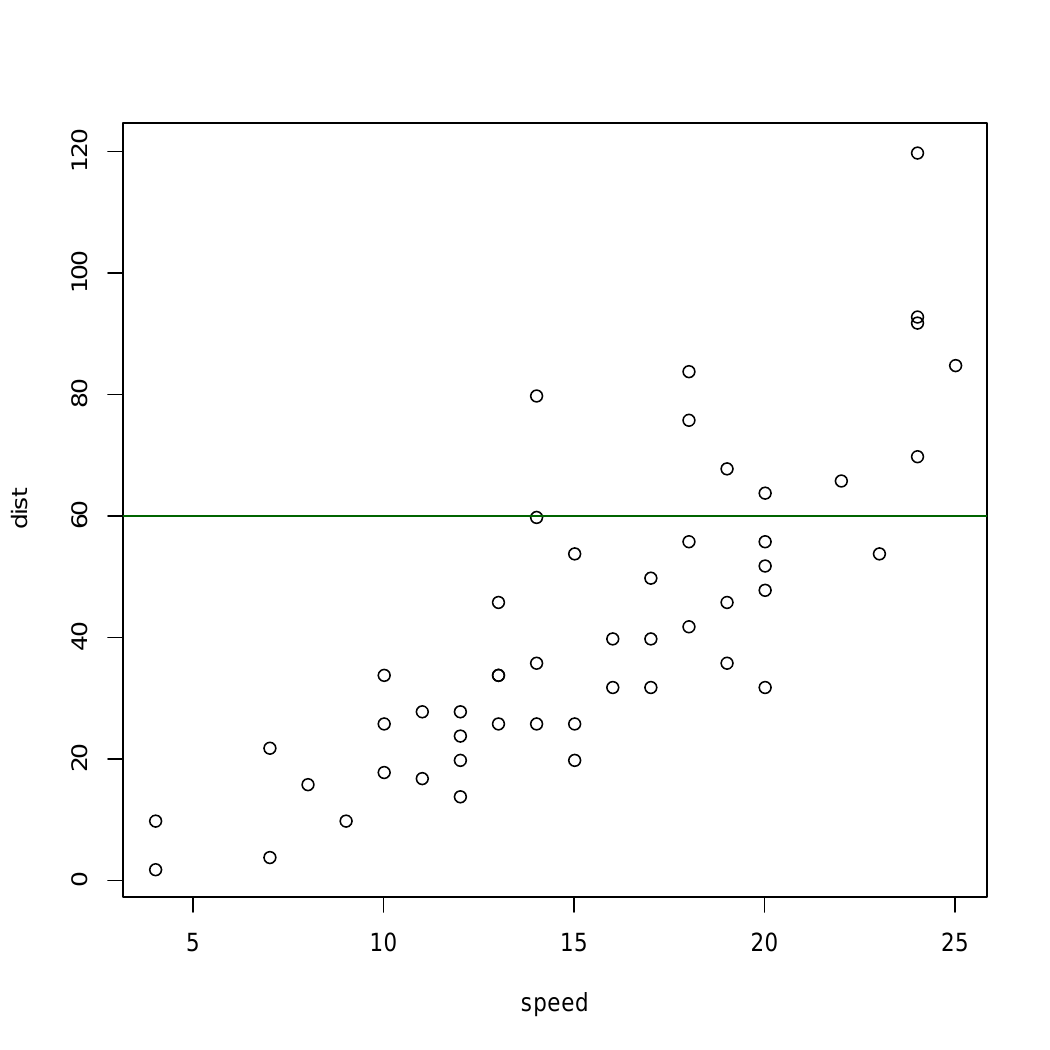In above example `abline()` Function draws an horizontal line on the current plot at the specified ‘x’ coordinates.

Example 3: To add a regression line

 `par``(mgp = ``c``(2, 1, 0), mar = ``c``(3, 3, 1, 1)) ` ` `  `# Fit regression line ` `require``(stats) ` `reg<-``lm``(dist ~ speed, data = cars) ` `coeff = ``coefficients``(reg) ` ` `  `# equation of the line :  ` `eq = ``paste0``(``"y = "``, ``round``(coeff, 1), ``"*x "``,  ` `                    ``round``(coeff, 1)) ` ` `  `# plot ` `plot``(cars, main = eq) ` `abline``(reg, col = ``"darkgreen"``) `

Output: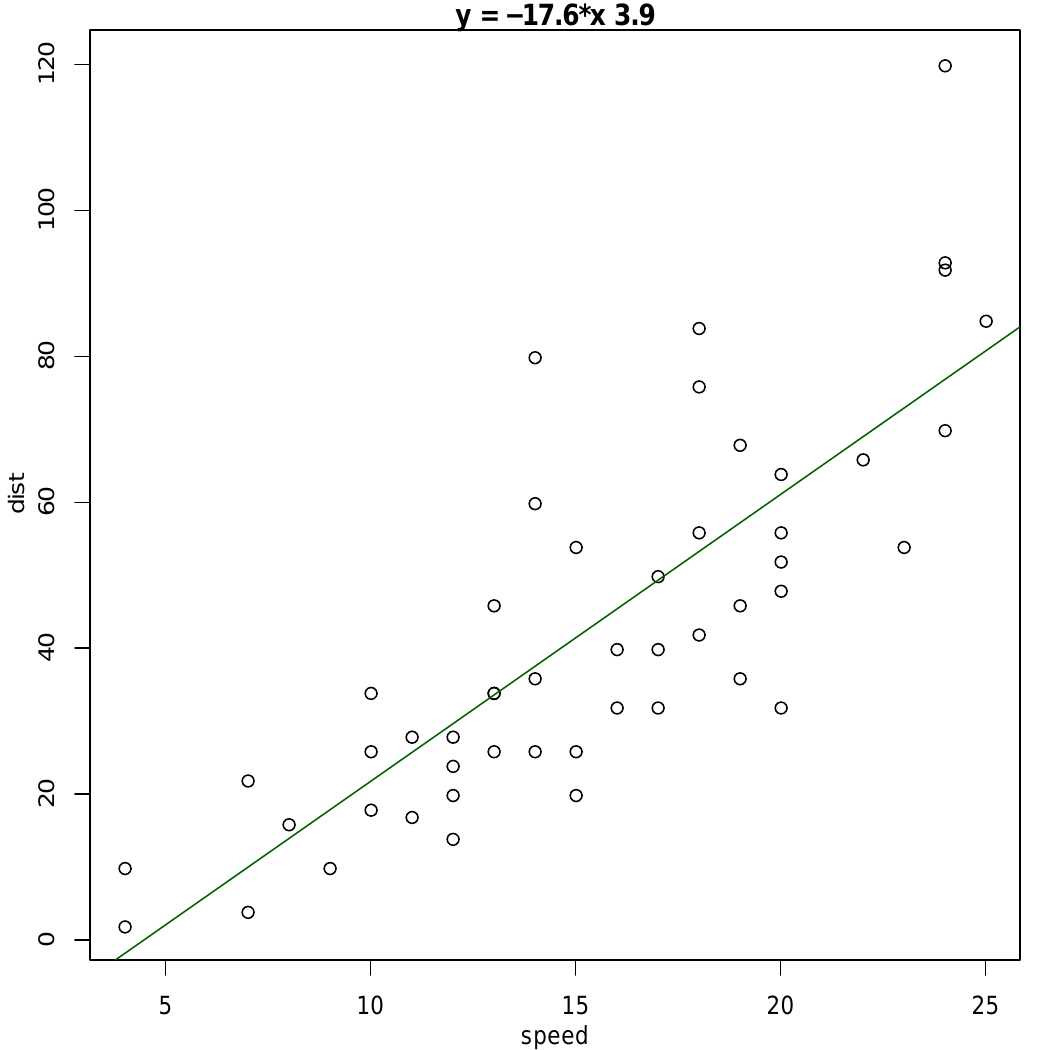In the above example, straight-line is added using the line equation and `abline()` function and plot relation between speed and distance.

My Personal Notes arrow_drop_upCheck out this Author's contributed articles.

If you like GeeksforGeeks and would like to contribute, you can also write an article using contribute.geeksforgeeks.org or mail your article to contribute@geeksforgeeks.org. See your article appearing on the GeeksforGeeks main page and help other Geeks.

Please Improve this article if you find anything incorrect by clicking on the "Improve Article" button below.

Article Tags :

Be the First to upvote.

Please write to us at contribute@geeksforgeeks.org to report any issue with the above content.